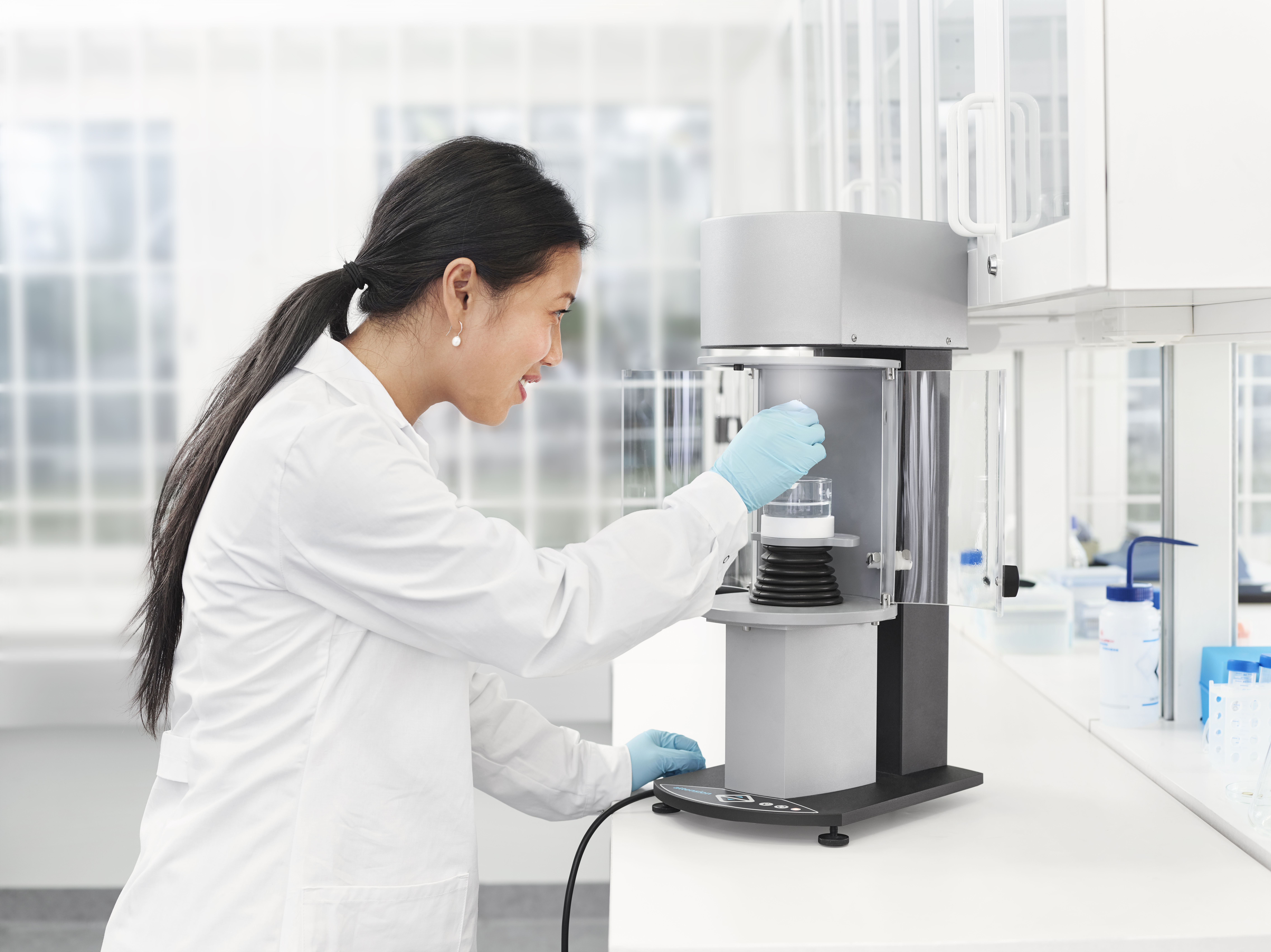Susanna Laurén Jan 5, ’21 > 5 min

# Wilhelmy plate method for contact angle measurement

## Surface Science Blog

Contact angle measurements are commonly used to evaluate the wettability of the surface. In addition to measurements done with the optical tensiometer, the force tensiometer offers an additional method for contact angle measurements; the Wilhelmy plate method.

## The basic principle of the Wilhelmy plate method

Wilhelmy plate refers to a thin plate with known dimensions, that is typically used to measure surface or interfacial tension between air-liquid or liquid-liquid interfaces, respectively. The same measurement principle can, however, also be used to measure the contact angle of a plate or a rod.

The Wilhelmy plate method is based on measuring the force F acting on the plate that is partially immersed into liquid. The force acting on the plate can be written as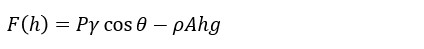, where P is the perimeter of the plate, γ is the surface tension of the liquid, θ is the contact angle between the plate and the measured liquid, ρ is the density of the liquid, A is the surface area of the plate, the immersion depth, and g the gravitational constant. The first term on the right-hand side of the equation is caused by wetting force and the second by buoyancy.

In the figure below, the force acting on the plate is illustrated at each step of the immersion cycle. First, the plate is hung on the balance and the measured force is caused by the mass of the plate. At the start of the measurement, the balance is typically zeroed (stage 1). When the plate touches the surface of the liquid that is automatically detected (stage 2). As the plate is immersed the force exerted on the plate is caused by the wetting force, the mass of the plate, and the buoyance (stage 3). The mass of the plate was already taken out by zeroing the balance.

The advancing and the receding angle can be solved by linear regression of the advancing and receding curves at zero depth, respectively.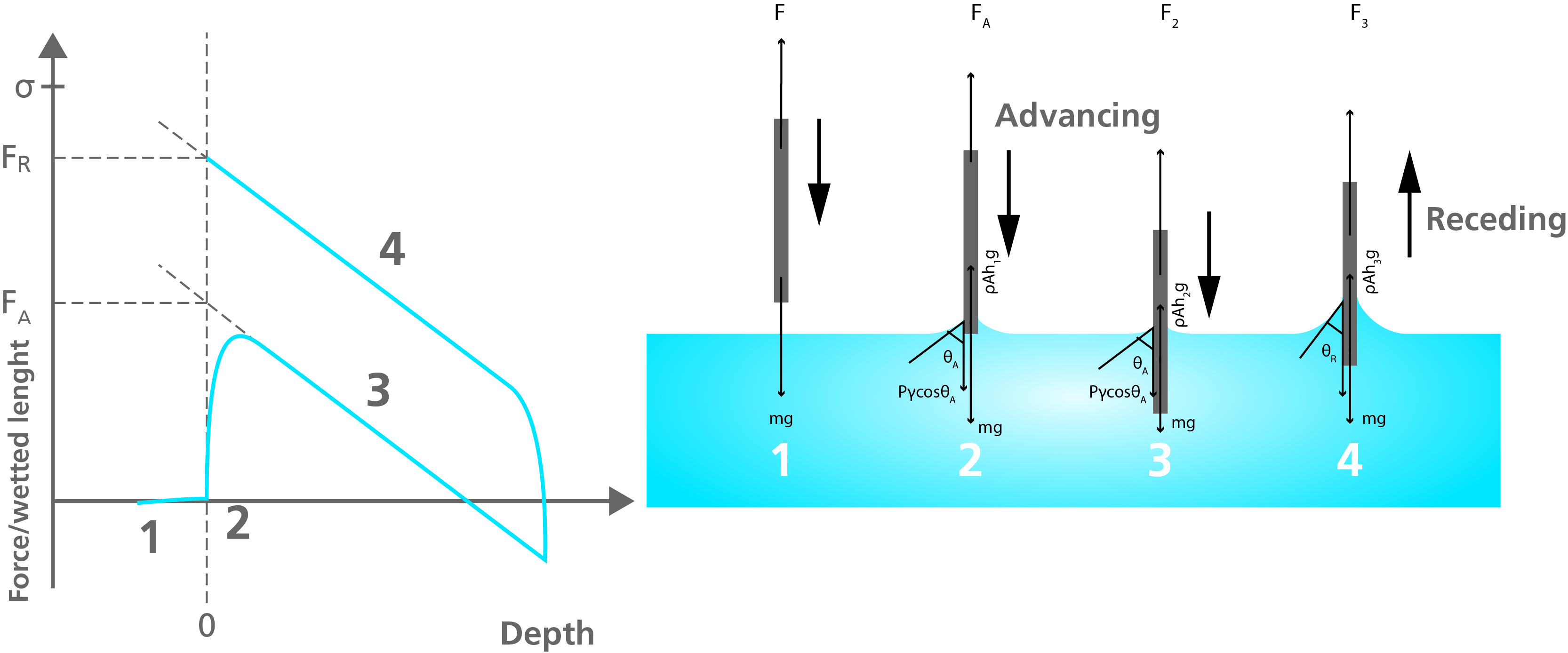When the Wilhelmy plate method is used for surface tension measurements, the plate is commonly made of platinum to ensure zero contact angle between the measured liquid and the plate. This leads to cosθ = 1, and the surface tension is then easily solved when the perimeter of the plate is known.

For contact angle measurements, the surface tension of the liquid needs to be known. Also, the perimeter of the sample, whether a plate or a rod needs to be known. Furthermore, the sample has to be uniform in shape through the whole immersion length, and homogenous on both sides of the samples as the contact angle is an average over the whole immersed area.

## Wilhelmy plate curves in practice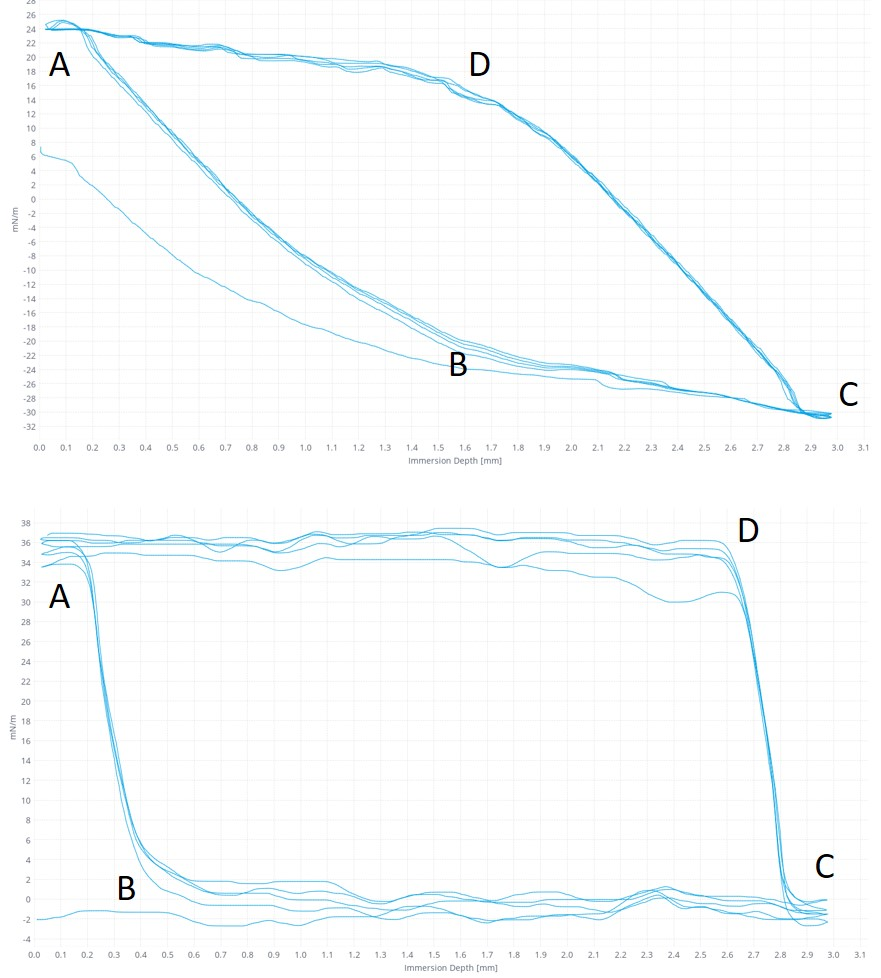In practice, the curves obtained with the Wilhelmy plate method typically look like the ones on the left. The data collection is started when the sample touches the liquid surface. This point is automatically detected by the software. The first part of the curve (AB) is not used in the contact angle analysis as the meniscus is still forming around the sample. When the meniscus is formed the advancing angle stabilizes. At the point C, the movement is reversed, and again some time is taken for the meniscus to stabilize (from C to D). The shape of the curve depends on the sample measured. The curve above is measured on a rectangular piece of polymer and the one below on thin fiber.

The Wilhelmy plate method gives the advancing and the receding contact angle as well as contact angle hysteresis for the immersed part of the sample.

If you would like to read more about Wilhelmy plate method and other contact angle measurement techniques, please download the white paper below## Related products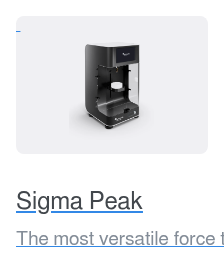## Explore the blog

You have only scratched the surface.